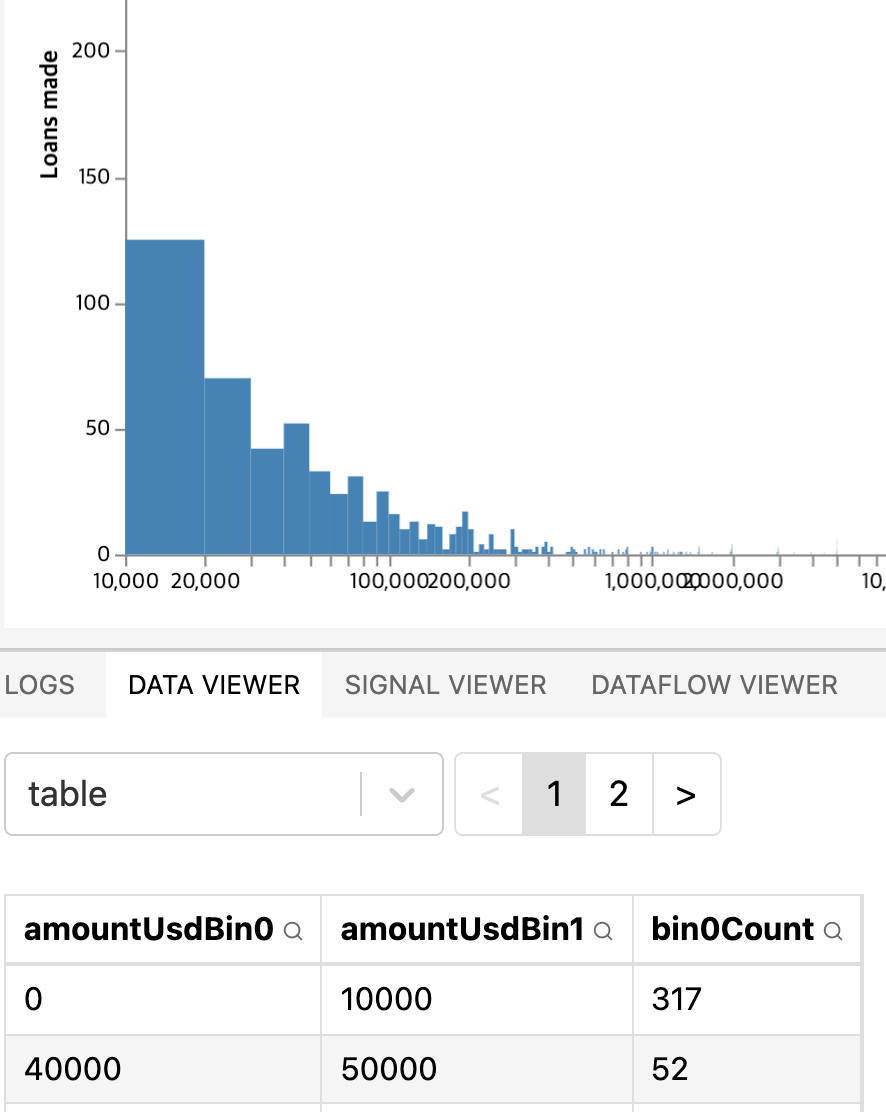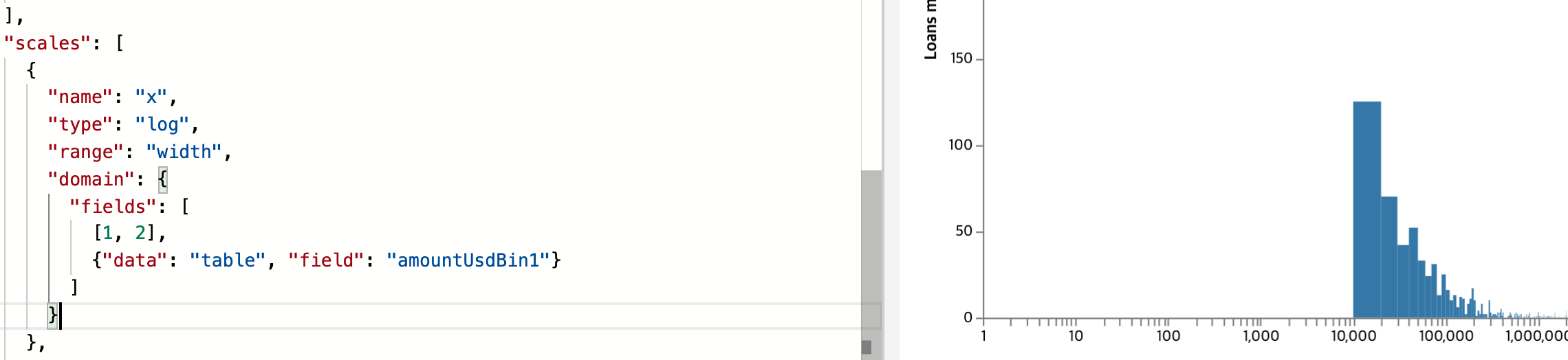# Part 7: Building a log-scaled histogram of Aave's loans in Vega

In the previous post, we built a pie chart to figure out the relative size of each coin. We figured precise percentages, and concluded that 5 coins were leading, and everything else accounted for less than 1% each. Let's stop looking at total volume for a moment and focus instead on the size of individual loans.

# Preparing the data

## Preparing bins

Using the bin transform doc, I started with this data definition:

"data": [
{
"name": "table",
"format": {"parse": {"date": "date"}},
"transform": [
{
"type": "bin",
"extent": [0, 1000000],
"field": "amountUsd",
"as": ["amountUsdBin0", "amountUsdBin1"]
}
],
"url": "https://gist.githubusercontent.com/atn832/9ec2c5629c91b09102c4d79dd72d0335/raw/0fc8878eb755e4828fd81a5315e956b413bf4ce1/1000%2520latest%2520Aave%2520loans.json"
}
],


What this does is assign a bin to each loan:

I had to hardcode the extend because it is a required field, and I haven't figured out how to make it compute the bounds automatically. Ideally, I'd just specify the number of bins, and it'd figure out the bounds for me.

The next step is to count how many loans are in each bin. For this we'll use an aggregate transform:

{
"type": "aggregate",
"ops": ["count"],
"groupby": ["amountUsdBin0", "amountUsdBin1"],
"as": ["bin0Count", "bin1Count"]
}


It looks like Infinity happens when the amountUsd is larger than the extent of my bins:

## Computing bin ranges

Let's try using the extent transform. This transform computes the bounds of a field, then creates a signal that contains [min, max]. Then we can pass the signal directly to the bin transform's extent field:

"transform": [
{"type": "extent", "field": "amountUsd", "signal": "amountUsdExtent"},
{
"type": "bin",
"extent": {"signal": "amountUsdExtent"},
"field": "amountUsd",
"as": ["amountUsdBin0", "amountUsdBin1"]
},
{
"type": "aggregate",
"ops": ["count"],
"groupby": ["amountUsdBin0", "amountUsdBin1"],
"as": ["bin0Count", "bin1Count"]
}
],


This fixes the Infinity issue. Now the issue is that the bins don't show meaningful information anymore. There's a bin that tells me that 99.1% of the loans are between 0 and $10m, and that there are a few outliers up to$150m.

By setting "maxbins": 500, I get barely more information:91% of loans are smaller than $500k. In my mind, I had expected a nice bell curve. However with bins of equal sizes, it won't happen. I could define the steps manually, and give a large interval to include large loans, and narrow intervals for smaller loans, since that's where most of the data points are grouped. But I can't think of an efficient way to define those steps. Or I could simply set one step, like "step": 10000,. With a step of$10k, I get somewhat meaningful numbers:

Let's plot this, then tweak the step to our liking.

# Plotting the bars

"marks": [
{
"type": "rect",
"from": {"data": "table"},
"encode": {
"enter": {
"x": {"scale": "x", "field": "amountUsdBin0"},
"x2": {"scale": "x", "field": "amountUsdBin1"},
"y": {"scale": "y", "value": 0},
"y2": {"scale": "y", "field": "bin0Count"},
"fill": {"value": "steelblue"}
}
}
}
]


I defined the bars like this. Here's what I got:

Isn't it an empty chart, you might ask. Not quite! Look at the bottom left corner:

What can we do to make it more visible? I could use a log scale for X. Let's try that. The problem is, log scales can't contain zero, yet my scale currently does contain zero because of the first amountUsdBin0:

"scales": [
{
"name": "x",
"type": "log",
"range": "width",
"domain": {
"fields": [
{"data": "table", "field": "amountUsdBin0"}
{"data": "table", "field": "amountUsdBin1"}
]
}
},


If I simply remove amountUsdBin0, then my very first bar won't show up anymore:If I omit amountUsdBin0 from the extent, I lose the first bar from 0 to $10k with the count of 317. Let's try to remove it and replace a hardcoded value of$1. The doc on multi-field data reference states the following about the fields property:

An array of basic data references indicating each data set and field value to include in the domain. In addition, array literals (e.g., [0, 100], ["a", "b", "c"]) may be included as elements of the fields array for inclusion in the domain determination.

So let's add an array litteral to it:Forcing the scale to start at 1 fixes the X axis, but the first bar is still not being rendered.